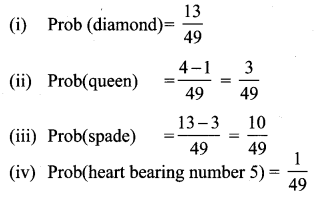# Samacheer Kalvi 10th Maths Solutions Chapter 8 Statistics and Probability Unit Exercise 8

## Tamilnadu Samacheer Kalvi 10th Maths Solutions Chapter 8 Statistics and Probability Unit Exercise 8

Question 1.
The mean of the following frequency distribution is 62.8 and the sum of all frequencies is 50. Compute the missing frequencies f1 and f2.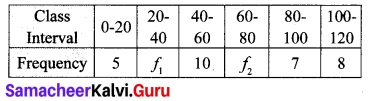Solution:
Mean $$\overline{x}$$ = 62.8
$$\Sigma x$$ = 50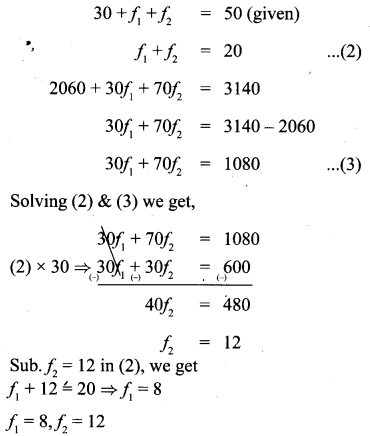Question 2.
The diameter of circles (in mm) drawn in a design are given below.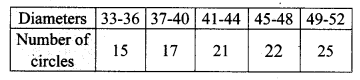Claculate the standard deviation.
Solution: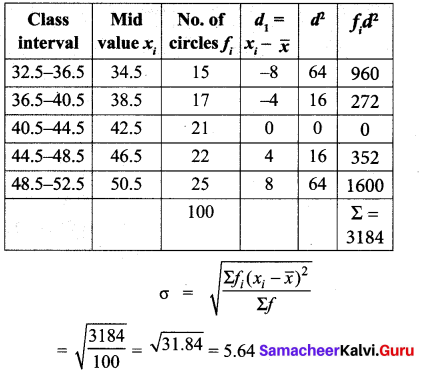Question 3.
The frequency distribution is given below.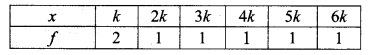In the table, k is a positive integer, has a varience of 160. Determine the value of k.
Solution: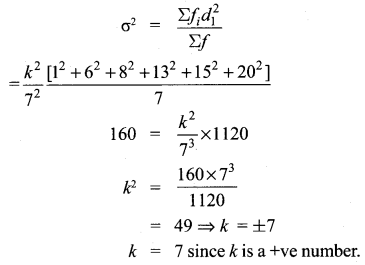Question 4.
The standard deviation of some temperature data in degree Celsius (°C) is 5. If the data were converted into degree Fahrenheit (°F) then what is the variance?
Standard deviation (σ) = 5
Variance = 52 = 25
We know the formula, F = $$\frac{9}{5}$$ C + 32
Variance (F) = Vanance $$\frac{9}{5}$$ C° + 32
[Variance of ax + b = a2 (variance of x)]
= $$\left(\frac{9}{5}\right)^{2}$$ . variance
= $$\frac{81}{25}$$ × 25
= 81° F
New variance = 81° FQuestion 5.
If for a distribution, $$\Sigma(x-5)=3, \Sigma(x-5)^{2}=43$$ and total number of observations is 18, find the mean and standard deviation.
Solution: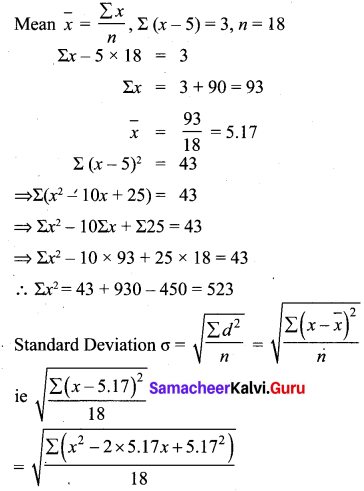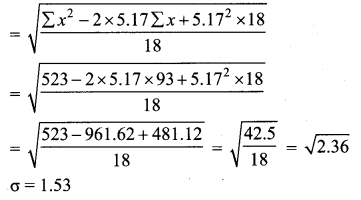Question 6.
Prices of peanut packets in various places of two cities are given below. In which city, prices were more stable?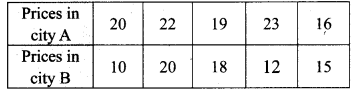Solution: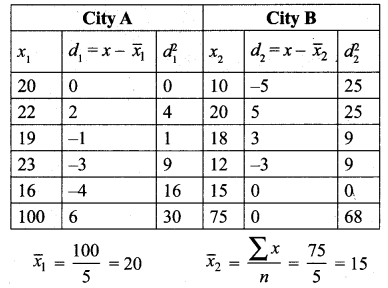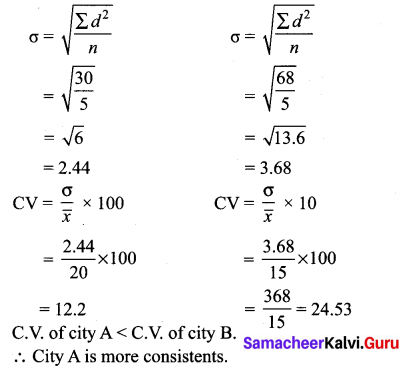Question 7.
If the range and coefficient of range of the data are 20 and 0.2 respectively, then find the largest and smallest values of the data.
Solution:
Range = L – S = 20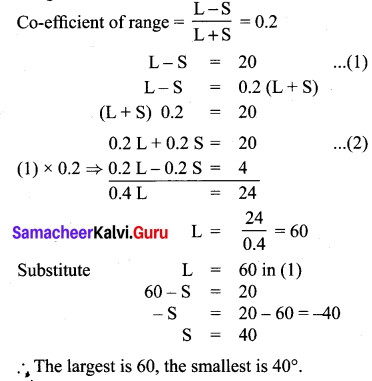Question 8.
If two dice are rolled, then find the probability of getting the product of face value 6 or the difference of face values 5.
Solution:
Product of face values 6: {(1, 6), (2, 3), (6, 1), (3,2)}
Difference of face value 5: {(1, 6), (6, 1)}Question 9.
In a two children family, find the probability that there is at least one girl in a family.
Solution:
S = {BB, BG, GB, GG}
n(S) = 4
Event of atleast one girl in a family say A
A= {BG, GB, GG}
n( A) = 3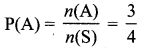Probability of at least one girl in a family is $$\frac{3}{4}$$Question 10.
A bag contains 5 white and some black balls. If the probability of drawing a black ball from the bag is twice the probability of drawing a white ball then find the number of black balls.
Solution:
Let a number of black balls be ‘x’.
Number of white balls = 5.Number of Black balls = 10.

Question 11.
The probability that a student will pass the final examination in both English and Tamil is 0.5 and the probability of passing neither is 0.1. If the probability of passing the English examination is 0.75, what is the probability of passing the Tamil examination?
Solution: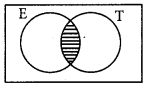P(English) = 0.75
P(Tamil) = x(assume)
P(English ∪ Tamil) = P(English) + P(Tamil) – P(English ∩ Tamil)
⇒ 1 – 0.1 = 0.75 + x – 0.5
⇒ x = 0.9 – 0.25Question 12.
The King, Queen and Jack of the suit spade are removed from a deck of 52 cards. One card is selected from the remaining cards. Find the probability of getting
(i) a diamond
(ii) a queen